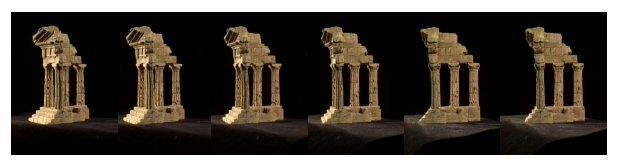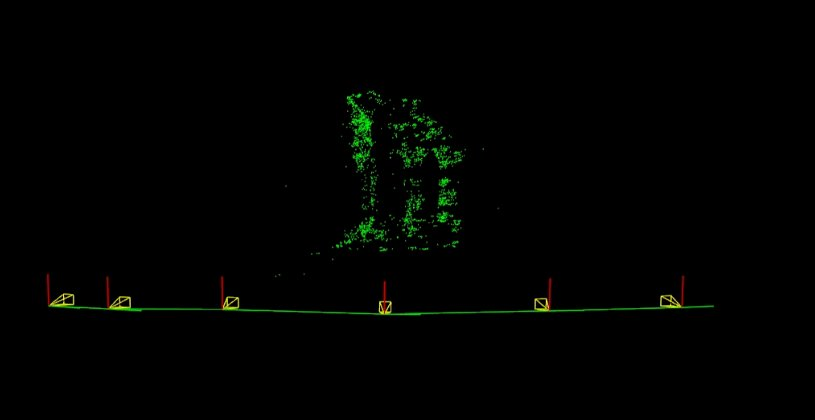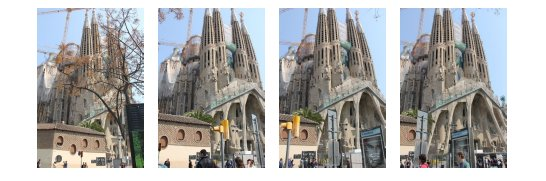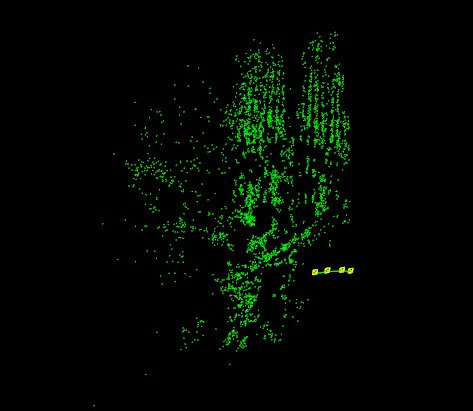OpenCV  4.5.3 Open Source Computer Vision
Scene Reconstruction

## Goal

In this tutorial you will learn how to use the reconstruction api for sparse reconstruction:

• Load and file with a list of image paths.
• Run libmv reconstruction pipeline.
• Show obtained results using Viz.

## Code

#include <opencv2/sfm.hpp>
#include <opencv2/viz.hpp>
#include <opencv2/core.hpp>
#include <iostream>
#include <fstream>
using namespace std;
using namespace cv;
using namespace cv::sfm;
static void help() {
cout
<< "\n------------------------------------------------------------------------------------\n"
<< " This program shows the multiview reconstruction capabilities in the \n"
<< " OpenCV Structure From Motion (SFM) module.\n"
<< " It reconstruct a scene from a set of 2D images \n"
<< " Usage:\n"
<< " example_sfm_scene_reconstruction <path_to_file> <f> <cx> <cy>\n"
<< " where: path_to_file is the file absolute path into your system which contains\n"
<< " the list of images to use for reconstruction. \n"
<< " f is the focal length in pixels. \n"
<< " cx is the image principal point x coordinates in pixels. \n"
<< " cy is the image principal point y coordinates in pixels. \n"
<< "------------------------------------------------------------------------------------\n\n"
<< endl;
}
static int getdir(const string _filename, vector<String> &files)
{
ifstream myfile(_filename.c_str());
if (!myfile.is_open()) {
cout << "Unable to read file: " << _filename << endl;
exit(0);
} else {;
size_t found = _filename.find_last_of("/\\");
string line_str, path_to_file = _filename.substr(0, found);
while ( getline(myfile, line_str) )
files.push_back(path_to_file+string("/")+line_str);
}
return 1;
}
int main(int argc, char* argv[])
{
if ( argc != 5 )
{
help();
exit(0);
}
// Parse the image paths
vector<String> images_paths;
getdir( argv, images_paths );
// Build intrinsics
float f = atof(argv),
cx = atof(argv), cy = atof(argv);
Matx33d K = Matx33d( f, 0, cx,
0, f, cy,
0, 0, 1);
bool is_projective = true;
vector<Mat> Rs_est, ts_est, points3d_estimated;
reconstruct(images_paths, Rs_est, ts_est, K, points3d_estimated, is_projective);
// Print output
cout << "\n----------------------------\n" << endl;
cout << "Reconstruction: " << endl;
cout << "============================" << endl;
cout << "Estimated 3D points: " << points3d_estimated.size() << endl;
cout << "Estimated cameras: " << Rs_est.size() << endl;
cout << "Refined intrinsics: " << endl << K << endl << endl;
cout << "3D Visualization: " << endl;
cout << "============================" << endl;
viz::Viz3d window("Coordinate Frame");
window.setWindowSize(Size(500,500));
window.setWindowPosition(Point(150,150));
window.setBackgroundColor(); // black by default
// Create the pointcloud
cout << "Recovering points ... ";
// recover estimated points3d
vector<Vec3f> point_cloud_est;
for (int i = 0; i < points3d_estimated.size(); ++i)
point_cloud_est.push_back(Vec3f(points3d_estimated[i]));
cout << "[DONE]" << endl;
cout << "Recovering cameras ... ";
vector<Affine3d> path;
for (size_t i = 0; i < Rs_est.size(); ++i)
path.push_back(Affine3d(Rs_est[i],ts_est[i]));
cout << "[DONE]" << endl;
if ( point_cloud_est.size() > 0 )
{
cout << "Rendering points ... ";
viz::WCloud cloud_widget(point_cloud_est, viz::Color::green());
window.showWidget("point_cloud", cloud_widget);
cout << "[DONE]" << endl;
}
else
{
cout << "Cannot render points: Empty pointcloud" << endl;
}
if ( path.size() > 0 )
{
cout << "Rendering Cameras ... ";
window.showWidget("cameras_frames_and_lines", viz::WTrajectory(path, viz::WTrajectory::BOTH, 0.1, viz::Color::green()));
window.showWidget("cameras_frustums", viz::WTrajectoryFrustums(path, K, 0.1, viz::Color::yellow()));
window.setViewerPose(path);
cout << "[DONE]" << endl;
}
else
{
cout << "Cannot render the cameras: Empty path" << endl;
}
cout << endl << "Press 'q' to close each windows ... " << endl;
window.spin();
return 0;
}

## Explanation

Firstly, we need to load the file containing list of image paths in order to feed the reconstruction api:

/home/eriba/software/opencv_contrib/modules/sfm/samples/data/images/resized_IMG_2889.jpg
/home/eriba/software/opencv_contrib/modules/sfm/samples/data/images/resized_IMG_2890.jpg
/home/eriba/software/opencv_contrib/modules/sfm/samples/data/images/resized_IMG_2891.jpg
/home/eriba/software/opencv_contrib/modules/sfm/samples/data/images/resized_IMG_2892.jpg
...
int getdir(const string _filename, vector<string> &files)
{
ifstream myfile(_filename.c_str());
if (!myfile.is_open()) {
cout << "Unable to read file: " << _filename << endl;
exit(0);
} else {
string line_str;
while ( getline(myfile, line_str) )
files.push_back(line_str);
}
return 1;
}

Secondly, the built container will be used to feed the reconstruction api. It is important outline that the estimated results must be stored in a vector<Mat>. In this case is called the overloaded signature for real images which from the images, internally extracts and compute the sparse 2d features using DAISY descriptors in order to be matched using FlannBasedMatcher and build the tracks structure.

bool is_projective = true;
vector<Mat> Rs_est, ts_est, points3d_estimated;
reconstruct(images_paths, Rs_est, ts_est, K, points3d_estimated, is_projective);
// Print output
cout << "\n----------------------------\n" << endl;
cout << "Reconstruction: " << endl;
cout << "============================" << endl;
cout << "Estimated 3D points: " << points3d_estimated.size() << endl;
cout << "Estimated cameras: " << Rs_est.size() << endl;
cout << "Refined intrinsics: " << endl << K << endl << endl;

Finally, the obtained results will be shown in Viz.

## Usage and Results

In order to run this sample we need to specify the path to the image paths files, the focal length of the camera in addition to the center projection coordinates (in pixels).

1. Middlebury temple

Using following image sequence  and the followings camera parameters we can compute the sparse 3d reconstruction:

./example_sfm_scene_reconstruction image_paths_file.txt 800 400 225The following picture shows the obtained camera motion in addition to the estimated sparse 3d reconstruction: